Aa Bb Cc Dd Ee Ff Gg Hh Ii Jj Kk Ll Mm Nn Oo Pp Qq Rr Ss Tt Uu Vv Ww Xx Yy Zz

Ii

interest

• interest is a fee paid for borrowing money or other assets.
• the amount borrowed is called the principal.
•  the interest is expressed as a percentage rate of the principal
for a given time interval.
• two common types of interest are simple interest
and compound interest

simple interest formula
I = P x R x T
I = Interest, P = Principle, R = Rate, T = Time

EXAMPLE: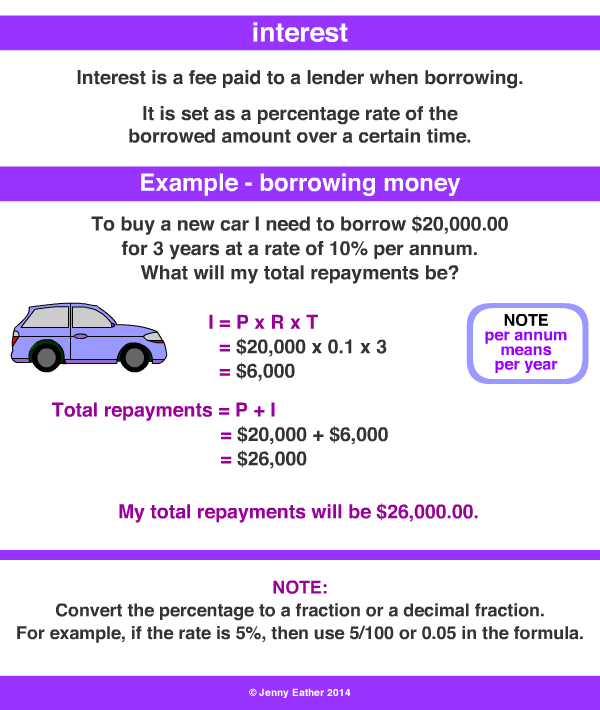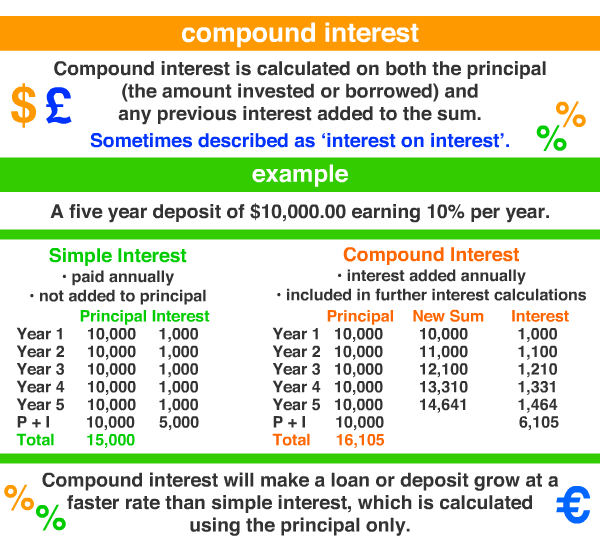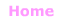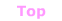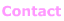© Jenny Eather 2014. All rights reserved.